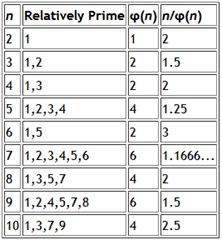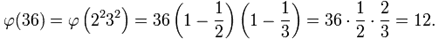# Project Euler – Problem 69 Solution

Presently sponsored by Serverless Guru: Your guide to cloud excellence, helping you every step of your serverless journey, including team training, pattern development, mass service migrations, architecting, and developing new solutions. Speak to a Guru today.

#### Problem

Euler’s Totient function, ?(n) [sometimes called the phi function], is used to determine the number of numbers less than n which are relatively prime to n. For example, as 1, 2, 4, 5, 7, and 8, are all less than nine and relatively prime to nine, ?(9)=6.It can be seen that n=6 produces a maximum n/?(n) for n <= 10.

Find the value of n <= 1,000,000 for which n/?(n) is a maximum.

#### Solution

```let hasDivisor(n) =
let upperBound = bigint(sqrt(double(n)))
[2I..upperBound] |> Seq.exists (fun x -> n % x = 0I)

let isPrime(n) = if n = 1I then false else not(hasDivisor(n))

// define the sequence of prime numbers
let primeSeq =
Seq.unfold (fun state -> if state >= 3I then Some(state, state+2I) else Some(state, state+1I)) 1I
|> Seq.filter isPrime
|> Seq.cache

// define function to find the prime denominators for a number n
let getPrimeFactors n =
let rec getPrimeFactorsRec denominators n =
if n = 1I then denominators
else
let denominator = primeSeq |> Seq.filter (fun x -> n % x = 0I) |> Seq.head
getPrimeFactorsRec (denominators @ [denominator]) (n/denominator)
getPrimeFactorsRec [] n

let totient n =
let primeFactors = getPrimeFactors n |> Seq.distinct
n * (primeFactors |> Seq.map (fun n' -> n'-1I) |> Seq.reduce (*)) / (primeFactors |> Seq.reduce (*))

let answer = [2I..1000000I] |> List.maxBy (fun n -> double(n) / double(totient n))
```

Having borrowed a number of functions I had used in previous solutions, the main function of interest here is the totient function. Going by the information on Euler’s totient function from wikipeidia and mathworld, the value of the totient function of n is be calculated using its prime factors:In words, this says that the distinct prime factors of 36 are 2 and 3; half of the thirty-six integers from 1 to 36 are divisible by 2, leaving eighteen; a third of those are divisible by 3, leaving twelve coprime to 36. And indeed there are twelve: 1, 5, 7, 11, 13, 17, 19, 23, 25, 29, 31, and 35.

Which in essence is what the totient function in my solution is doing, except to avoid any rounding errors it actually multiplies the number n by the product of the numerators before dividing by the product of denominators, i.e. 36 * (1 * 2) / (2 * 3) instead of 36 * 0.5 * 0.66666666667 which introduces a rounding error which may or may not has an influence in the result.

#### Update 2011/11/06:

This solution takes a long time and doesn’t comply with the under one minute rule, by using a prime sieve like this one I was able to get the execution time down to under 50s on my machine.  Here is the revised code using the aforementioned sieve implementation:

Enjoy!

### 11 thoughts on “Project Euler – Problem 69 Solution”

1.any thoughts about how to get the time for that down to under a minute?

2.Jorge – most of the time is spent in the prime number generation, if you use a prime sieve like this one (http://blog.hoomla.se/post/The-sieve-of-Atkin-in-F.aspx) you should be able to get the time down to under a minute.

I’ll post a revised solution which runs in under 50s on my machine.

3.This line seems awfully inefficent:

let pFact = primeNumbers |> List.find (fun n’ -> n % n’ = 0) // This seems awfully inefficient.

If n is prime (and very large), won’t you be doing lots of divisions, just to find out that n is prime?

I am just learning f#, so thanks for posting your solution. Very helpful.

4.Jorge – you’re right, you can further optimize by taking care of the case where n is prime by building up a cache of the prime numbers, I’ve updated the solution which now runs in 8 seconds. Thanks for the suggestion!

5.Wow. Nice optimization!

One thing I have been wondering about — maybe you can help: I’d like to keep adding useful functions to my “ProjectEuler.fs” library. But this GetPrimeFactors rec function is hard to “reuse” because it depends on isPrime, which depends on the cache object.

Any thoughts about how to package this all up for reuse? Or is that just not the F# way?

6.Jorge – how about putting everything prime-related into a PrimeHelper class and add a Initialize(max : int) method that initializes a cache of prime numbers up to max? That way, you can then reuse GetPrimeFactors and other prime-related functions with a handy cache which you’ll initialize hopefully only first.

Additionally, you might want to add protection against isPrime because called with a value that’s outside your primes range, etc. which can be something as simple as:

type PrimeHelper() =
let mutable max = 0

member this.Initialize n =
// …initialize cache
// set max after you’re done
max <- n member this.IsPrime n = if n > max then this.Initialize n
// …use cache

Modules are good for prototyping and quickly putting together something that ‘works’, but on the long run and especially for reusability I prefer to convert them to a class at some point, which also makes them easier to use in other .Net languages.

7.Thanks for that tip, too.

8.1/2(6n-(-1)^n+3)= 5,7,11,13,17,19,23,25,29,31,35,37,41. n={1,2,3,4,…} It eliminates all multiples of 3 so we are left with primes, primes*primes and powers of primes*primes. its a feedback loop. 3 is a special case prime in this because its the only prime with a digital root 3. Thats why I leave it out along with 1 and 2. basically the function is saying, starting on 5 add 2 then add 4 then add 2,+4+2+4+2+4….

9.I’ve not found any primes that have a digital root of 3,6 or 9, maybe I’m wrong didn’t go to in depth with that one, other then 3